The NLIN Procedure

PROC NLIN Statement

• PROC NLIN <options>;

The PROC NLIN statement invokes the NLIN procedure.

Table 81.1 summarizes the options available in the PROC NLIN statement. All options are subsequently discussed in alphabetical order.

Table 81.1: Summary of Options in PROC NLIN Statement

Option

Description

Options Related to Data Sets

Specifies the input data set

Specifies the output data set for parameter estimates, covariance matrix, and so on

Requests that final estimates be added to the OUTEST= data set

Optimization Options

Limits display of grid search

Chooses the optimization method

Specifies the maximum number of iterations

Specifies the maximum number of step halvings

Allows the objective function to increase between iterations

Controls the step-size search

Specifies the step-size search method

Controls the step-size search

Uses the Moore-Penrose inverse

Does not expense degrees of freedom when bounds are active

Specifies the fixed value for residual variance

Singularity and Convergence Criteria

Tunes the convergence criterion

Uses the change in loss function as the convergence criterion and tunes its value

Uses the maximum change in parameter estimates as the convergence criterion and tunes its value

Tunes the singularity criterion used in matrix inversions

ODS Graphics Options

Produces ODS graphical displays

Displayed Output

Adds Hougaard’s skewness measure to the "Parameter Estimates" table

Adds Box’s bias measure to the "Parameter Estimates" table

Suppresses the "Iteration History" table

Suppresses displayed output

Displays the model program and variable list

Selects the LIST , LISTDEP , LISTDER , and LISTCODE options

Displays the model program code

Displays dependencies of model variables

Displays the derivative table

Displays the global nonlinearity measures table

Adds the uncorrected or corrected total sum of squares to the analysis of variance table

Displays the cross-reference of variables

Trace Model Execution

Displays execution messages for program statements

Displays results of statements in model program

Displays results of operations in model program

ALPHA=
specifies the level of significanceused in the construction of% confidence intervals. The value must be strictly between 0 and 1; the default value of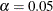results in 95% intervals. This value is used as the default confidence level for limits computed in the "Parameter Estimates" table and with the LCLM , LCL , UCLM , and UCL options in the OUTPUT statement.

BEST=n

requests that PROC NLIN display the residual sums of squares only for the best n combinations of possible starting values from the grid. If you do not specify the BEST= option, PROC NLIN displays the residual sum of squares for every combination of possible parameter starting values.

BIAS

adds Box’s bias and percentage bias measures to the "Parameter Estimates" table (Box 1971). Box’s bias measure, along with Hougaard’s measure of skewness, is used for assessing a parameter estimator’s close-to-linear behavior (Ratkowsky 1983, 1990). Hence, it is useful for identifying problematic parameters (Seber and Wild 1989, sec. 4.7.1). When you specify the BIAS option, Box’s bias measure (Box 1971) and the percentage bias (the bias expressed as a percentage of the least-squares estimator) are added for each parameter to the "Parameter Estimates" table. Ratkowsky (1983, p. 21) takes a percentage bias in excess of 1% to be a good rule of thumb for indicating nonlinear behavior.

See the section Box’s Measure of Bias for further details. Example 81.4 shows how to use this measure, along with Hougaard’s measure of skewness, to evaluate changes in the parameterization of a nonlinear model. Computation of the Box’s bias measure requires first and second derivatives. If you do not provide derivatives with the DER statement—and it is recommended that you do not—the analytic derivatives are computed for you.

CONVERGE=c

specifies the convergence criterion for PROC NLIN. For all iterative methods the relative offset convergence measure of Bates and Watts is used by default to determine convergence. This measure is labeled "R" in the "Estimation Summary" table. The iterations are said to have converged for CONVERGE=c if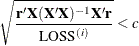whereis the residual vector andis the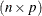matrix of first derivatives with respect to the parameters. The default LOSS function is the sum of squared errors (SSE), and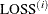denotes the value of the loss function at the ith iteration. By default, CONVERGE=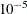. The R convergence measure cannot be computed accurately in the special case of a perfect fit (residuals close to zero). When the SSE is less than the value of the SINGULAR= criterion, convergence is assumed.

CONVERGEOBJ=c

uses the change in the LOSS function as the convergence criterion and tunes the criterion. The iterations are said to have converged for CONVERGEOBJ=c if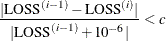where LOSS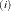is the LOSS for the ith iteration. The default LOSS function is the sum of squared errors (SSE), the residual sum of squares. The constant c should be a small positive number. For more details about the LOSS function, see the section Special Variable Used to Determine Convergence Criteria. For more details about the computational methods in the NLIN procedure, see the section Computational Methods.

Note that in SAS 6 the CONVERGE= and CONVERGEOBJ= options both requested that convergence be tracked by the relative change in the loss function. If you specify the CONVERGEOBJ= option in newer releases, the CONVERGE= option is disabled. This enables you to track convergence as in SAS 6.

CONVERGEPARM=c

uses the maximum change among parameter estimates as the convergence criterion and tunes the criterion. The iterations are said to have converged for CONVERGEPARM=c if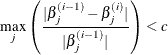where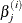is the value of the jth parameter at the ith iteration.

The default convergence criterion is CONVERGE . If you specify CONVERGEPARM=c, the maximum change in parameters is used as the convergence criterion. If you specify both the CONVERGEOBJ= and CONVERGEPARM= options, PROC NLIN continues to iterate until the decrease in LOSS is sufficiently small (as determined by the CONVERGEOBJ= option) and the maximum change among the parameters is sufficiently small (as determined by the CONVERGEPARM= option).

DATA=SAS-data-set

specifies the input SAS data set to be analyzed by PROC NLIN. If you omit the DATA= option, the most recently created SAS data set is used.

FLOW

displays a message for each statement in the model program as it is executed. This debugging option is rarely needed, and it produces large amounts of output.

G4

uses a Moore-Penrose inverse (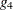-inverse) in parameter estimation. See Kennedy and Gentle (1980) for details.

HOUGAARD

adds Hougaard’s measure of skewness to the "Parameter Estimates" table (Hougaard 1982, 1985). The skewness measure is one method of assessing a parameter estimator’s close-to-linear behavior in the sense of Ratkowsky (1983, 1990). The behavior of estimators that are close to linear approaches that of least squares estimators in linear models, which are unbiased and have minimum variance. When you specify the HOUGAARD option, the standardized skewness measure of Hougaard (1985) is added for each parameter to the "Parameter Estimates" table. Because of the linkage between nonlinear behavior of a parameter estimator in nonlinear regression and the nonnormality of the estimator’s sampling distribution, Ratkowsky (1990, p. 28) provides the following rules to interpret the (standardized) Hougaard skewness measure:

• Values less than 0.1 in absolute value indicate very close-to-linear behavior.

• Values between 0.1 and 0.25 in absolute value indicate reasonably close-to-linear behavior.

• The nonlinear behavior is apparent for absolute values above 0.25 and is considerable for absolute values above 1.

See the section Hougaard’s Measure of Skewness for further details. Example 81.4 shows how to use this measure to evaluate changes in the parameterization of a nonlinear model. Computation of the Hougaard skewness measure requires first and second derivatives. If you do not provide derivatives with the DER statement—and it is recommended that you do not—the analytic derivatives are computed for you. For weighted least squares, the NLIN procedure ignores the weights for computing the Hougaard skewness measure. This can be a strong assumption as the formulation in Hougaard (1985) assumes homoscedastic errors.

LIST

displays the model program and variable lists, including the statements added by macros. Note that the expressions displayed by the LIST option do not necessarily represent the way the expression is actually calculated—because intermediate results for common subexpressions can be reused—but are shown in expanded form. To see how the expression is actually evaluated, use the LISTCODE option.

LISTALL

selects the LIST , LISTDEP , LISTDER , and LISTCODE options.

LISTCODE

displays the derivative tables and the compiled model program code. The LISTCODE option is a debugging feature and is not normally needed.

LISTDEP

produces a report that lists, for each variable in the model program, the variables that depend on it and the variables on which it depends.

LISTDER

displays a table of derivatives. The derivatives table lists each nonzero derivative computed for the problem. The derivative listed can be a constant, a variable in the model program, or a special derivative variable created to hold the result of an expression.

MAXITER=n

specifies the maximum number n of iterations in the optimization process. The default is n = 100.

MAXSUBIT=n

places a limit on the number of step halvings. The value of MAXSUBIT must be a positive integer and the default value is n = 30.

METHOD=GAUSS | MARQUARDT | NEWTON | GRADIENT

specifies the iterative method employed by the NLIN procedure in solving the nonlinear least squares problem. The GAUSS, MARQUARDT, and NEWTON methods are more robust than the GRADIENT method. If you omit the METHOD= option, METHOD=GAUSS is used. See the section Computational Methods for more information.

NLINMEASURES

displays the global nonlinearity measures table. These measures include the maximum intrinsic and parameter-effects curvatures (Bates and Watts 1980), the root mean square (RMS) intrinsic and parameter-effects curvatures and the critical curvature value (Bates and Watts 1980). In addition, the variances of the ordinary and projected residuals are included. According to Bates and Watts (1980), both intrinsic and parameter-effects curvatures are deemed negligible if they are less than the critical curvature value. This critical value is given by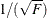where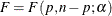. The value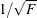can be considered as the radius of curvature of thepercent confidence region (Bates and Watts 1980). For weighted least squares, the NLIN procedure ignores the weights for computing the curvature measures. This can be a strong assumption as the original derivation in Bates and Watts (1980) assumes homoscedastic errors.

NOITPRINT

suppresses the display of the "Iteration History" table.

NOHALVE

removes the restriction that the objective value must decrease at every iteration. Step halving is still used to satisfy BOUNDS and to ensure that the number of observations that can be evaluated does not decrease. The NOHALVE option can be useful in weighted nonlinear least squares problems where the weights depend on the parameters, such as in iteratively reweighted least squares (IRLS) fitting. See Example 81.2 for an application of IRLS fitting.

NOPRINT

suppresses the display of the output. Note that this option temporarily disables the Output Delivery System (ODS). For more information, see Chapter 20: Using the Output Delivery System.

OUTEST=SAS-data-set

specifies an output data set that contains the parameter estimates produced at each iteration. See the section Output Data Sets for details. If you want to create a SAS data set in a permanent library, you must specify a two-level name. For more information about permanent libraries and SAS data sets, see SAS Language Reference: Concepts.

PLOTS <(global-plot-option)> <= (plot-request<(options)> <... plot-request<(options)>>)>

controls most of the plots that are produced through ODS Graphics (other plots are controlled by the BOOTSTRAP and PROFILE statements). When you specify only one plot-request, you can omit the parentheses around it. Here are some examples:

  plots
plots        = none
plots        = diagnostics(unpack)
plots        = fit(stats=none)
plots        = residuals(residualtype=proj unpack smooth)
plots(stats=all)  = (diagnostics(stats=(maxincurv maxpecurv)) fit)


ODS Graphics must be enabled before plots can be requested. For example:

ods graphics on;

proc nlin plots=diagnostics(stats=all);
model y = alpha - beta*(gamma**x);
run;

ods graphics off;


For more information about enabling and disabling ODS Graphics, see the section Enabling and Disabling ODS Graphics in Chapter 21: Statistical Graphics Using ODS.

If ODS Graphics is enabled and if you specify the PLOTS option without any global-plot-option or plot-requests, PROC NLIN produces the plots listed in Table 81.2 with the default set of statistics and options. If you do not specify the PLOTS option, PROC NLIN does not produce any of these graphs.

Table 81.2: Graphs Produced When the PLOTS Option Is Specified

Plot

Conditional On

ContourFitPlot

Model with two regressors

FitDiagnosticsPanel

Unconditional

FitPlot

Model with one regressor

LeveragePlot

Unconditional

LocalInfluencePlot

Unconditional

ResidualPanel

Unconditional

You can request additional plots by specifying plot-requests. For a listing of all the plots that PROC NLIN produces, see the section ODS Graphics. Each global-plot-option applies to all plots that are generated by the NLIN procedure except for plots that are controlled by the BOOTSTRAP and PROFILE statements. The global-plot-option can be overridden by a specific option after a plot-request.

The following global-plot-options are available:

RESIDUALTYPE=RAW | PROJ | BOTH

specifies the residual type to be plotted in the fit diagnostics and residual plots. RESIDUALTYPE=RAW requests that only the ordinary residuals be included in the plots; RESIDUALTYPE=PROJ sets the choice to projected residuals. By default, both residual types are included, which can also be effected by setting RESIDUALTYPE=BOTH. See the section Residuals in Nonlinear Regression for details about the properties of ordinary and projected residuals in nonlinear regression.

STATS=ALL | DEFAULT | NONE | (plot-statistics)

requests the statistics to be included in all plots, except the ResidualPlots and the unpacked diagnostics plots. Table 81.3 lists the statistics that you can request. STATS=ALL requests all these statistics, STATS=NONE suppresses all statistics, and STATS=DEFAULT selects the default statistics. You request statistics in addition to the default set by including the keyword DEFAULT in the plot-statistics list.

Table 81.3: Statistics Available in Plots

Keyword

Default

Description

DEFAULT

All default statistics

MAXINCURV

Maximum intrinsic curvature

MAXPECURV

Maximum parameter-effects curvature

MSE

x

Mean squared error, estimated or set by the SIGSQ option

NOBS

x

Number of observations used

NPARM

x

Number of parameters in the model

PVAR

x

Estimated variance of the projected residuals

RMSINCURV

Root mean square intrinsic curvature

RMSPECURV

Root mean square parameter-effects curvature

VAR

x

Estimated variance of the ordinary residuals

Along with the maximum intrinsic and parameter-effects curvatures, the critical curvature (CURVCRIT) value,where, is also displayed. You do not need to specify any option for it. See the section Relative Curvature Measures of Nonlinearity for details about curvature measures of nonlinearity.

UNPACK

suppresses paneling.

You can specify the following plot-requests in the PLOTS= option:

ALL

produces all appropriate plots.

NONE

suppresses all plots.

DIAGNOSTICS <(diagnostics-options)>

produces a summary panel of fit diagnostics, leverage plots, and local-influence plots. The fit diagnostics panel includes:

• histogram of the ordinary residuals

• histogram of the projected residuals

• response variable values versus the predicted values

• expectation or mean of the ordinary residuals versus the predicted values

• ordinary and projected residuals versus the predicted values

• standardized ordinary and projected residuals versus the predicted values

• standardized ordinary and projected residuals versus the tangential leverage

• standardized ordinary and projected residuals versus the Jacobian leverage

• box plot of the ordinary and projected residuals if you specify the STATS=NONE suboption

The leverage and local influence plots are produced separately. The leverage plot is an index plot of the tangential and Jacobian leverages (by observation), and the local-influence plot contains the local influence by observation for a perturbation of the response variable. See the sections Leverage in Nonlinear Regression and Local Influence in Nonlinear Regression for a some details about leverages and local-influence in nonlinear regression.

You can specify the following diagnostics-options:

RESIDUALTYPE=RAW | PROJ | BOTH

specifies the residual type to be plotted in the panel. See the RESIDUALTYPE= global-plot-option for details. This diagnostics-option overrides the PLOTS RESIDUALTYPE global-plot-option. Only the plots that overlay both ordinary and projected residuals in the same plot are affected by this option.

LEVERAGETYPE=TAN | JAC | BOTH

specifies the leverage type to be plotted in the leverage plot. LEVERAGETYPE=TAN specifies that only the tangential leverage be included in the leverage plot, and LEVERAGETYPE=JAC specifies that only the Jacobian leverage be included. By default, both are displayed in the leverage plot. The same result can be effected by setting LEVERAGETYPE=BOTH. Only the leverage plot is affected by this option.

LABELOBS

specifies that the leverage and local-influence plots be labeled with the observation number. Only these two plots are affected by this option.

STATS=stats-options

determines which statistics are included in the panel. See the STATS= global-plot-option for details. This diagnostics-option overrides the PLOTS STATS global-plot-option.

UNPACK

produces the plots in the diagnostics panel as individual plots. The statistics panel is not included in the individual plots, even if STATS= global-plot-option or STATS= diagnostics-option or both are specified.

FITPLOT | FIT <(fit-options)>

produces, depending on the number of regressors, a scatter or contour fit plot. For a single-regressor model, a scatter plot of the data overlaid with the regression curve, confidence, and prediction bands is produced. For two-regressor models, a contour fit plot of the model with overlaid data is produced. If the model contains more than two regressors, no fit plot is produced.

You can specify the following fit-options:

NOCLI

suppresses the prediction limits for single-regressor models.

NOCLM

suppresses the confidence limits for single-regressor models.

NOLIMITS

suppresses the confidence and prediction limits for single-regressor models.

controls how the observations are displayed. The suboptions are as follows:

specifies that observations be displayed as circles colored by the observed response. The same color gradient is used to display the fitted surface and the observations. Observations for which the predicted response is close to the observed response have similar colors—the greater the contrast between the color of an observation and the surface, the larger the residual is at that point. OBS=GRADIENT is the default.

NONE

suppresses the observations.

OUTLINE

specifies that observations be displayed as circles with a border but with a completely transparent fill.

is the same as OBS=GRADIENT except that a border is shown around each observation. This option is useful for identifying the location observations for which the residuals are small, because at these points the color of the observations and the color of the surface are indistinguishable.

CONTLEG

specifies that a continuous legend be included in the contour fit plot of a two-regressor model.

STATS=stats-options

determines which model fit statistics are included in the panel. See the STATS= global-plot-option for details. This fit-option overrides the PLOTS STATS global-plot-option.

RESIDUALS <(residual-options)>

produces panels of the ordinary and projected residuals versus the regressors in the model. Each panel contains at most six plots, and multiple panels are used in the case where there are more than six regressors in the model.

The following residual-options are available:

RESIDUALTYPE=RAW | PROJ | BOTH

specifies the residual type to be plotted in the panel. See the RESIDUALTYPE= global-plot-option for details. This residual-option overrides the PLOTS RESIDUALTYPE global-plot-option.

SMOOTH

requests a nonparametric smooth of the residuals for each regressor. Each nonparametric fit is a loess fit that uses local linear polynomials, linear interpolation, and a smoothing parameter selected that yields a local minimum of the corrected Akaike information criterion (AICC). See Chapter 71: The LOESS Procedure, for details.

UNPACK

suppresses paneling.

PRINT

displays the result of each statement in the program as it is executed. This option is a debugging feature that produces large amounts of output and is normally not needed.

RHO=value

specifies a value that controls the step-size search. By default RHO=0.1, except when METHOD= MARQUARDT. In that case, RHO=10. See the section Step-Size Search for more details.

SAVE

specifies that, when the iteration limit is exceeded, the parameter estimates from the final iteration be output to the OUTEST= data set. These parameter estimates are associated with the observation for which _TYPE_="FINAL". If you omit the SAVE option, the parameter estimates from the final iteration are not output to the data set unless convergence has been attained.

SIGSQ=value

specifies a value to use as the estimate of the residual variance in lieu of the estimated mean-squared error. This value is used in computing the standard errors of the estimates. Fixing the value of the residual variance can be useful, for example, in maximum likelihood estimation.

SINGULAR=s

specifies the singularity criterion, s, which is the absolute magnitude of the smallest pivot value allowed when inverting the Hessian or the approximation to the Hessian. The default value is 1E4 times the machine epsilon; this product is approximately 1E-12 on most computers.

SMETHOD=HALVE | GOLDEN | CUBIC

specifies the step-size search method. The default is SMETHOD=HALVE. See the section Step-Size Search for details.

TAU=value

specifies a value that is used to control the step-size search. The default is TAU=1, except when METHOD= MARQUARDT. In that case the default is TAU=0.01. See the section Step-Size Search for details.

TOTALSS

adds to the analysis of variance table the uncorrected total sum of squares in models that have an (implied) intercept, and adds the corrected total sum of squares in models that do not have an (implied) intercept.

TRACE

displays the result of each operation in each statement in the model program as it is executed, in addition to the information displayed by the FLOW and PRINT options. This debugging option is needed very rarely, and it produces even more output than the FLOW and PRINT options.

XREF

displays a cross-reference of the variables in the model program showing where each variable is referenced or given a value. The XREF listing does not include derivative variables.

UNCORRECTEDDF

specifies that no degrees of freedom be lost when a bound is active. When the UNCORRECTEDDF option is not specified, an active bound is treated as if a restriction were applied to the set of parameters, so one parameter degree of freedom is deducted.